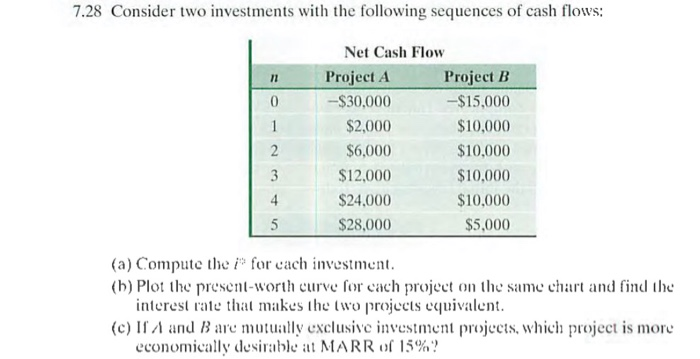# Question Solved1 Answer7.28 Consider two investments with the following sequences of cash flows: 0 Net Cash Flow Project A Project B -$30,000 -$15,000 $2,000$10,000 $6,000$10,000 $12,000$10,000 $24,000$10,000 $28.000$5,000 (a) Compute the iº for cach investment. (b) Plot the present worth curve for each project on the same chart and find the interest rate that makes the two projects equivalent. (c) If A and B are mutually exclusive investment projects, which project is more economically desirable at MARR of 15%?Transcribed Image Text: 7.28 Consider two investments with the following sequences of cash flows: 0 Net Cash Flow Project A Project B -$30,000 -$15,000 $2,000$10,000 $6,000$10,000 $12,000$10,000 $24,000$10,000 $28.000$5,000 (a) Compute the iº for cach investment. (b) Plot the present worth curve for each project on the same chart and find the interest rate that makes the two projects equivalent. (c) If A and B are mutually exclusive investment projects, which project is more economically desirable at MARR of 15%?
More
Transcribed Image Text: 7.28 Consider two investments with the following sequences of cash flows: 0 Net Cash Flow Project A Project B -$30,000 -$15,000 $2,000$10,000 $6,000$10,000 $12,000$10,000 $24,000$10,000 $28.000$5,000 (a) Compute the iº for cach investment. (b) Plot the present worth curve for each project on the same chart and find the interest rate that makes the two projects equivalent. (c) If A and B are mutually exclusive investment projects, which project is more economically desirable at MARR of 15%?